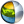Cg 3.1 Toolkit Documentation Cg / Standard Library / normalize

## Name

normalize - normalizes a vector

## Synopsis

```float normalize(float v);
float normalize(float1 v);
float normalize(float2 v);
float normalize(float3 v);
float normalize(float4 v);

half normalize(half v);
half normalize(half1 v);
half normalize(half2 v);
half normalize(half3 v);
half normalize(half4 v);

fixed normalize(fixed v);
fixed normalize(fixed1 v);
fixed normalize(fixed2 v);
fixed normalize(fixed3 v);
fixed normalize(fixed4 v);
```

## Parameters

v
Vector to normalize.

## Description

Returns the normalized version of a vector, meaning a vector in the same direction as the original vector but with a Euclidean length of one.

## Reference Implementation

normalize for a float3 vector could be implemented like this.

```float3 normalize(float3 v)
{
return rsqrt(dot(v,v))*v;
}
```

## Profile Support

normalize is supported in all profiles except fp20.| Cg Toolkit | Download | Release Archive | Profiles | Reference | Books | Discussions |